# An exhaustive Python cheat sheet 1/2

You’ll find below a summary of the most important key features of the Python programming language. Its clear syntax is illustrated trough simple examples.

I’ve made an other cheat sheet with a focus on the Object Oriented part of Python and on functionnal programming. You can find all of this content in a dedicated anki deck to help you memorizing it.

Cards are composed of a simple challenge, then answer shows the code and its’ result in a dedicated jupyter notebook also available on github.

## Design

what are the characteristics of the Python programming language ?

• interpreted
• high-level
• philosophy emphasizes on code readability (indentation)
• object-oriented
• dynamically-typed
• garbage-collected
• structured (procedural)
• functional programming
• described as a “batteries included”

## Definitions

Explain what means “interpreted” ?
An interpreter is a computer program that directly executes instructions written in a programming or scripting language, without requiring them previously to have been compiled into a machine language program.

Explain what means “high-level” ?
A high-level programming language is a programming language with strong abstraction from the details of the computer.

Explain what means “object-oriented” ?
Object Oriented programming (OOP) is a programming paradigm that relies on the concept of classes and objects. It is used to structure a software program into simple, reusable pieces of code blueprints (usually called classes), which are used to create individual instances of objects.

Explain what means “dynamically-typed” ?
This means that the Python interpreter does type checking only as code runs, and the type of a variable is allowed to change over its lifetime.

Explain what means “garbage-collected” ?
Garbage collection (GC) is a form of automatic memory management. The garbage collector attempts to reclaim memory which was allocated by the program, but is no longer referenced—also called garbage.

Explain what means “structured (procedural)” ?
Structured programming is a programming paradigm aimed at improving the clarity, quality, and development time by making extensive use of the structured control flows and repetition, block structures. Procedural programming is an otherparadigm based on the concept of the routine or subroutine(series of computational steps).

Explain what means “functional programming” ?
Functional programming is a paradigm where programs are constructed by applying and composing functions (rather than a sequence of imperative statements which update the running state of the program).

Explain why Python is described as “batteries included” ?
This is due to its comprehensive standard library

## Basic Types

Boolean
What are the values of a boolean variable ?

``````True
False
``````
``````False
``````

Integers
Integer values - all kinds, also in binary or hex

``````0
-192
0b010
0xF3
``````
``````243
``````

Strings
a basic string

``````st = "One\tTwo\nThree"
st
``````
``````'One\tTwo\nThree'
``````
``````print(st)
``````
``````One	Two
Three
``````

a multiple line string

``````st = """multiple
lines
long
string"""

st
``````
``````'multiple \nlines\nlong\nstring'
``````
``````print(st)
``````
``````multiple
lines
long
string
``````

a string with a single quote inside

``````st = "I'm"
print(st)
``````
``````I'm
``````

escape a single quote in a string

``````st = 'I\'m'
print(st)
``````
``````I'm
``````

Lists
an empty list

``````[]
``````
``````[]
``````

a list with various values

``````['x', 11, 8.9]
``````
``````['x', 11, 8.9]
``````

a list with a single element

``````['st']
``````
``````['st']
``````

Tuples
an empty tuple

``````()
``````
``````()
``````

a tuple with various elements

``````11, 'y', 7.4
``````
``````(11, 'y', 7.4)
``````

a tuple with a single element

``````('st')
``````
``````'st'
``````

Dictionnary
an empty dictionnary

``````{}
``````
``````{}
``````

a dictionnary with 2 keys

``````{1: 'one', 'two': 2}
``````
``````{1: 'one', 'two': 2}
``````

Set
a set with various elements

``````{1, 1, 2, 3}
``````
``````{1, 2, 3}
``````

Type conversion
convert a float to an integer

``````int(1.2)
``````
``````1
``````

convert an integer to a float

``````float(1)
``````
``````1.0
``````

convert an integer to a boolean of value True

``````bool(1)
``````
``````True
``````
``````bool(2)
``````
``````True
``````

convert an integer to a boolean of value False

``````bool(0)
``````
``````False
``````

convert an integer to a string

``````str(123)
``````
``````'123'
``````

convert a boolean to a string

``````str(True)
``````
``````'True'
``````

convert a set to a list

``````list({1 ,2, 3})
``````
``````[1, 2, 3]
``````

convert a string to a list

``````list("adfs")
``````
``````['a', 'd', 'f', 's']
``````

convert a list to a string

``````my_list = ['I', "'", 'l', 'u', 'v']
my_list
``````
``````['I', "'", 'l', 'u', 'v']
``````
``````''.join(my_list)
``````
``````"I'luv"
``````

Iterables objects - properties

/ List Tuple Set Dict
symbol [,] (,) {,} {k: v,}
type ordered ordered unordered unordered
access index index value key
property mutable immutable unique values keys/values

## Assignements

multiple assignement of the same value to 3 different variables

``````a = b = c = 0
a +=1
a, b
``````
``````(1, 0)
``````

assign 2 different values to 2 differents variables in one line

``````a, b = 1, 2
a, b
``````
``````(1, 2)
``````

swap 2 variables’ values

``````a, b = 2, 8
a, b = b, a
a, b
``````
``````(8, 2)
``````

list unpacking

``````x, *y, z = (10, 'a', 'b', 20)
x, z
``````
``````(10, 20)
``````

string unpacking

``````i, j = 'ab'
i, j
``````
``````('a', 'b')
``````

get the last line of a log

``````*content, last_line = open('/etc/fstab')
last_line
``````
``````'# UNCONFIGURED FSTAB FOR BASE SYSTEM\n'
``````

decrement a variable

``````a = 0
a -= 1
a
``````
``````-1
``````

find the remainder of a division

``````a = 16
a %= 5
a
``````
``````1
``````

find the result of a division

``````a = 16
a /= 5
a
``````
``````3.2
``````

calculate the quotient of a division

``````a = 16
a //= 5
a
``````
``````3
``````

delete a variable

``````a = 0
del a
a
``````
``````---------------------------------------------------------------------------

NameError                                 Traceback (most recent call last)

<ipython-input-46-c1e82bdf26d0> in <module>()
1 a = 0
2 del a
----> 3 a

NameError: name 'a' is not defined
``````

delete a user-defined objects, lists etc

``````a, l = 1, [1, 2, 3]
del a, l
a, l
``````
``````---------------------------------------------------------------------------

NameError                                 Traceback (most recent call last)

<ipython-input-10-4d62949f80bf> in <module>
1 a, l = 1, [1, 2, 3]
2 del a, l
----> 3 a, l

NameError: name 'a' is not defined
``````

delete items within lists, dictionaries

``````l = [1, 2, 3]
del l
l
``````
``````[1, 3]
``````

last value used by the interpreter

``````10
``````
``````10
``````
``````_
``````
``````10
``````

ignore a specific value

``````a, _, b = (1, 2, 3)
_
``````
``````2
``````

ignore specific values

``````a, *_, b = (1, 2, 3, 4, 5)
_
``````
``````[2, 3, 4]
``````

## Calculation / Operators

”+ - * / ** % // ~”

complementary

``````~1
``````
``````-2
``````

check if two objects are the same object:

``````a = 'test'
b = a
a is b
``````
``````True
``````

test if two objects that are equal, but not the same object:

``````x = ["apple", "banana"]
y = ["apple", "banana"]
x is y
``````
``````False
``````
``````x is not y
``````
``````True
``````
``````x = y
x is y
``````
``````True
``````

test if two objects are not the same object:

``````a = 'test'
b = 'TEST'
a is not b
``````
``````True
``````

test if an element is in a list

``````'a' in ['c', 'b', 'a']
``````
``````True
``````

test if an element is not in a list

``````'z' not in ['c', 'b', 'a']
``````
``````True
``````

the opposite of True

``````not True
``````
``````False
``````

Or condition with 2 booleans

``````True or False
``````
``````True
``````

And condition with 2 booleans

``````True and True
``````
``````True
``````

## Misc Operators

Source w3schools

### Python Arithmetic Operators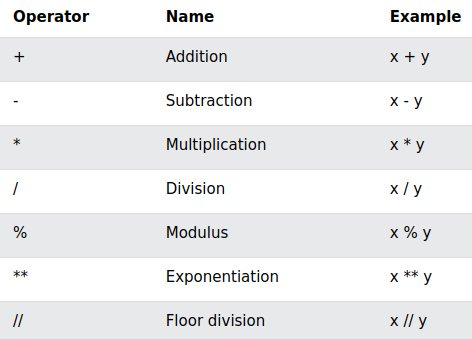### Python Assignment Operators### Python Comparison Operators### Python Logical Operators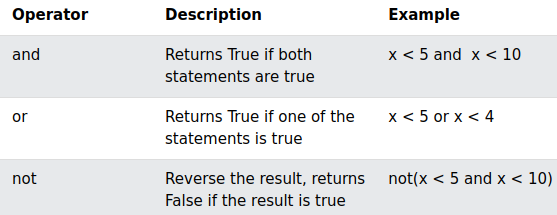### Python Identity Operators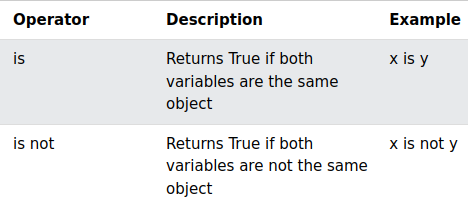### Python Membership Operators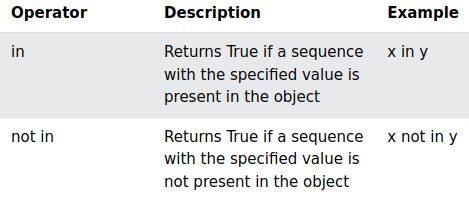### Python Bitwise Operators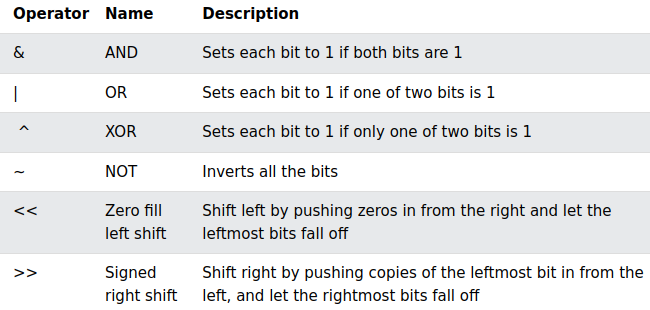``````0b0011 & 0b1100
``````
``````0
``````

or bitwise operator

``````0b0011 | 0b1100
``````
``````15
``````

Shift left by pushing zeros in from the right and let the leftmost bits fall off

``````0b0001 << 1
``````
``````2
``````

Inverts all the bits

``````~ 0b0001
``````
``````-2
``````

Sets each bit to 1 if only one of two bits is 1

``````0b0001 ^ 0b0000
``````
``````1
``````

test if 2 variables are equal

``````a, b = 10, 10.0
a == b
``````
``````True
``````

test if 2 variables are different

``````a, b = 1, 2
a != b
``````
``````True
``````
``````0 == False
``````
``````True
``````
``````# everything not equals to 0 is True
0 != True
``````
``````True
``````

Python Operator Precedence From Python documentation on operator precedence (Section 5.15)

Highest precedence at top, lowest at bottom. Operators in the same box evaluate left to right.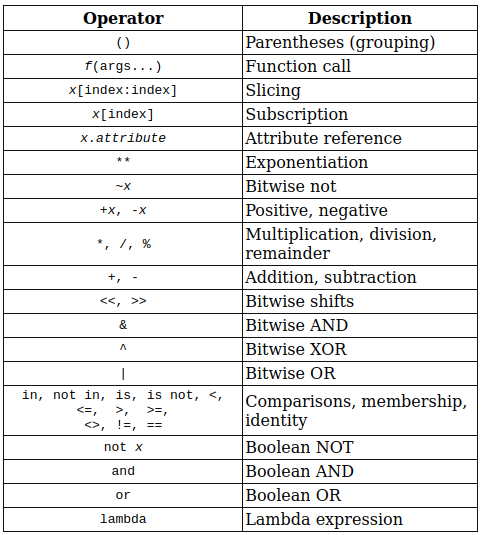concatenate 2 lists

``````['a', 'b'] + [1, 2]
``````
``````['a', 'b', 1, 2]
``````

concatenate strings

``````'this' + 'is' 'hot'
``````
``````'thisishot'
``````

repeat the same string three times

``````'test_' * 3
``````
``````'test_test_test_'
``````

repeat the same list three times

``````[1, 2, 3] * 3
``````
``````[1, 2, 3, 1, 2, 3, 1, 2, 3]
``````

loop on the indexes & values of a list

``````lst = ['a', 'b', 'c']
for i, j in enumerate(lst):
print(f'index {i} & value {j}')
``````
``````index 0 & value a
index 1 & value b
index 2 & value c
``````

list integers from 0 to 10

``````range(10)
``````
``````range(0, 10)
``````
``````list(range(10))
``````
``````[0, 1, 2, 3, 4, 5, 6, 7, 8, 9]
``````

list integers from 2 to 5

``````list(range(2, 6))
``````
``````[2, 3, 4, 5]
``````

list integers with a step of 2

``````list(range(2, 7, 2))
``````
``````[2, 4, 6]
``````

## Containers Ops

get the min value of a list

``````lst = [1, 2, 3]
min(lst)
``````
``````1
``````

get the max value of a list

``````lst = [1, 2, 3]
max(lst)
``````
``````3
``````

get the sum of all values of a list

``````lst = [1, 2, 3]
sum(lst)
``````
``````6
``````

get the length of a list

``````lst = [1, 2, 3, 4]
len(lst)
``````
``````4
``````

test if an element is in a list

``````lst = [1, 2, 3]
1 in lst
``````
``````True
``````
``````lst = [1, 2, 3]
4 in lst
``````
``````False
``````

get a sorted list

``````lst = [3, 1, 2, 3]
sorted(lst)
``````
``````[1, 2, 3, 3]
``````

get a list of tuples from 2 list of the same lenght

``````lst_1 = [1, 2, 3]
lst_2 = ['a', 'b', 'c']
list(zip(lst_1, lst_2))
``````
``````[(1, 'a'), (2, 'b'), (3, 'c')]
``````

check if all the value of a list are True

``````lst = [True, True, True]
all(lst)
``````
``````True
``````
``````lst = [False, True, True]
all(lst)
``````
``````False
``````

check if at least one value of a list is True

``````lst = [False, False, True]
any(lst)
``````
``````True
``````
``````lst = [False, False, False]
any(lst)
``````
``````False
``````

reverse a list

``````lst = [3, 1, 2, 3]
list(reversed(lst))
``````
``````[3, 2, 1, 3]
``````
``````lst[::-1]
``````
``````[3, 2, 1, 3]
``````

repeat the same string three times

``````s = "string__"
s * 3
``````
``````'string__string__string__'
``````

concatenate two strings

``````"string___" + " " "test"
``````
``````'string___ test'
``````

get the index of an element

``````lst = [3, 1, 2, 3]
lst.index(1)
``````
``````1
``````
``````lst = [3, 1, 2, 3]
lst.index(3)
``````
``````0
``````

count the number of an element

``````lst = [3, 1, 2, 3]
lst.count(1)
``````
``````1
``````
``````lst = [3, 1, 2, 3]
lst.count(3)
``````
``````2
``````

## List Operators

add an element at the end of a list

``````lst = [3, 1, 2, 3]
lst.append(0)
lst
``````
``````[3, 1, 2, 3, 0]
``````

remove the 2nd element of a list

``````lst = [3, 1, 2, 3]
lst.remove(1)
lst
``````
``````[3, 2, 3]
``````

reverse the elements of a list in the same variable

``````lst = [4, 1, 2, 3]
lst.reverse()
lst
``````
``````[3, 2, 1, 4]
``````

sort the elements of a list in the same variable

``````lst = [4, 1, 2, 3]
lst.sort()
lst
``````
``````[1, 2, 3, 4]
``````
``````lst = [4, 1, 2, 3]
sorted(lst)
``````
``````[1, 2, 3, 4]
``````
``````lst
``````
``````[4, 1, 2, 3]
``````

add a list at the end of an other list

``````lst = [4, 1, 2, 3]
lst.extend([0, 0])
lst
``````
``````[4, 1, 2, 3, 0, 0]
``````

add an element at the beginning of a list

``````lst = [4, 1, 2, 3]
lst.insert(0, 7)
lst
``````
``````[7, 4, 1, 2, 3]
``````

add an element at the 2nd place of a list

``````lst = [4, 1, 2, 3]
lst.insert(1, 7)
lst
``````
``````[4, 7, 1, 2, 3]
``````

add an element at the end of a list (with insert or plus)

``````lst = [4, 1, 2, 3]
lst.insert(-1, 7)
lst
``````
``````[4, 1, 2, 7, 3]
``````
``````[4, 1, 2, 3] + 
``````
``````[4, 1, 2, 3, 7]
``````

retrieve the 2nd element of a list and delete it

``````lst = [4, 7, 1, 2, 3]
lst.pop(1)
``````
``````7
``````
``````lst
``````
``````[4, 1, 2, 3]
``````

## Dict Operators

get the value of a corresponding dict key

``````d = {'a': 1, 'b': 2, 'c': 3}
d['a']
``````
``````1
``````

list all keys of a dict

``````d = {'a': 1, 'b': 2, 'c': 3}
d.keys()
``````
``````dict_keys(['a', 'b', 'c'])
``````

list all values of a dict

``````d = {'a': 1, 'b': 2, 'c': 3}
d.values()
``````
``````dict_values([1, 2, 3])
``````

list tuples (k, v) of a dict

``````d = {'a': 1, 'b': 2, 'c': 3}
d.items()
``````
``````dict_items([('a', 1), ('b', 2), ('c', 3)])
``````

get the value of a corresponding dict key (with a default one)

``````d = {'a': 1, 'b': 2, 'c': 3}
d.get('a', 7)
``````
``````1
``````
``````d = {'a': 1, 'b': 2, 'c': 3}
d.get('z', 7)
``````
``````7
``````

get the key of the min value in a dict

``````d = {'a': 1, 'b': 2, 'c': 0}
min(d, key=d.get)
``````
``````'c'
``````

removes the item that was last inserted into the dict & returns it

``````d = {'a': 1, 'b': 2, 'c': 3}
d.popitem()
``````
``````('c', 3)
``````
``````d
``````
``````{'a': 1, 'b': 2}
``````

removes & returns an item in the dict

``````d = {'a': 1, 'b': 2, 'c': 3}
d.pop('b')
``````
``````2
``````
``````d
``````
``````{'a': 1, 'c': 3}
``````

## String Operators

convert a string in uppercase

``````st = "abcdefgh"
st.upper()
``````
``````'ABCDEFGH'
``````
``````st
``````
``````'abcdefgh'
``````

convert a string in lowercase

``````st = "ABCDEFGH"
st.lower()
``````
``````'abcdefgh'
``````

put a capital at the beginning of a string

``````st = "this is a test"
st.capitalize()
``````
``````'This is a test'
``````

replace a char by an other in a string

``````st = "abcdefgh"
st.replace('e', 'Z')
``````
``````'abcdZfgh'
``````
``````st = "EabcdEfghE"
st.replace('E', 'Z')
``````
``````'ZabcdZfghZ'
``````

remove the spaces at the beginning / the end of a string

``````st = "  this is a test  "
st.strip(' ')
``````
``````'this is a test'
``````

create a title from a string

``````st = "this is a test"
st.title()
``````
``````'This Is A Test'
``````

split lines in a string

``````st = "this is a 1st line\nthis is a 2nd line"
print(st)
print(st.splitlines())
``````
``````this is a 1st line
this is a 2nd line
['this is a 1st line', 'this is a 2nd line']
``````

use an f_string with a float

``````v = 3.14159
f'value of Pi: {v:.2f}'
``````
``````'value of Pi: 3.14'
``````

test if a string contains only letters

``````name = "Monica"
name.isalpha()
``````
``````True
``````
``````# contains whitespace
name = "Monica Geller"
name.isalpha()
``````
``````False
``````
``````# contains number
name = "Mo3nicaGell22er"
name.isalpha()
``````
``````False
``````

test if a string contains only letters & numbers

``````name = "Mo3nicaGell22er"
name.isalnum()
``````
``````True
``````
``````name = "Mo3nica Gell22er"
name.isalnum()
``````
``````False
``````

## Loops

a simple for loop

``````for i in range(0, 3):
print(i**3)
``````
``````0
1
8
``````

a simple while loop

``````i = 0
while i < 4:
print(i**3)
i += 1
``````
``````0
1
8
27
``````

a while loop with conditions to break / continue

``````i = 0
while i < nb:
if cond1:
break
elif cond2:
continue
else:
pass
i += 1
``````

get user input

``````st = input("Enter your name: ")
print(f"You're name is {st}", "test", sep="---", end="___")
``````
``````Enter your name: Me
You're name is Me---test___
``````

## Comprehensions

a list comprehension to get even number with only a if (no elese)

``````[i for i in range(10) if i%2 == 0]
``````
``````[0, 2, 4, 6, 8]
``````

a list comprehension to list even/odd number (with if and else cases)

``````["Even" if i%2==0 else "Odd" for i in range(10)]
``````
``````['Even', 'Odd', 'Even', 'Odd', 'Even', 'Odd', 'Even', 'Odd', 'Even', 'Odd']
``````

a dictionnary comprehension

``````d = {1: 10, 2: 20, 3: 30}
{k**2: v+1 for (k, v) in d.items()}
``````
``````{1: 11, 4: 21, 9: 31}
``````

reverse keys/values with a comprehension

``````d = {1: 10, 2: 20, 3: 30}
{v: k for (k, v) in d.items()}
``````
``````{10: 1, 20: 2, 30: 3}
``````

with a comprehension get a set of squared element from a list

``````lst = [1, 2, 3, 3, 2, 1]
{i**2 for i in lst}
``````
``````{1, 4, 9}
``````

## Case, switch statement

a switch statement with default value

``````def zero():
return "ZERO"

def one():
return "ONE"

def two():
return "TWO"

switcher = {
0: zero,
1: one,
2: two
}

def numbers_to_strings(argument):
"""Returns the func from switcher dic"""
func = switcher.get(argument, "nothing")
return func()

numbers_to_strings(1)
``````
``````'ONE'
``````
``````#changing the switch case
switcher=two

numbers_to_strings(1)
``````
``````'TWO'
``````
``````switcher()
``````
``````'ZERO'
``````

## Exceptions

Python Built-in Exceptions

``````print(dir(locals()['__builtins__']))
``````
``````['ArithmeticError', 'AssertionError', 'AttributeError', 'BaseException', 'BlockingIOError', 'BrokenPipeError', 'BufferError', 'BytesWarning', 'ChildProcessError', 'ConnectionAbortedError', 'ConnectionError', 'ConnectionRefusedError', 'ConnectionResetError', 'DeprecationWarning', 'EOFError', 'Ellipsis', 'EnvironmentError', 'Exception', 'False', 'FileExistsError', 'FileNotFoundError', 'FloatingPointError', 'FutureWarning', 'GeneratorExit', 'IOError', 'ImportError', 'ImportWarning', 'IndentationError', 'IndexError', 'InterruptedError', 'IsADirectoryError', 'KeyError', 'KeyboardInterrupt', 'LookupError', 'MemoryError', 'ModuleNotFoundError', 'NameError', 'None', 'NotADirectoryError', 'NotImplemented', 'NotImplementedError', 'OSError', 'OverflowError', 'PendingDeprecationWarning', 'PermissionError', 'ProcessLookupError', 'RecursionError', 'ReferenceError', 'ResourceWarning', 'RuntimeError', 'RuntimeWarning', 'StopAsyncIteration', 'StopIteration', 'SyntaxError', 'SyntaxWarning', 'SystemError', 'SystemExit', 'TabError', 'TimeoutError', 'True', 'TypeError', 'UnboundLocalError', 'UnicodeDecodeError', 'UnicodeEncodeError', 'UnicodeError', 'UnicodeTranslateError', 'UnicodeWarning', 'UserWarning', 'ValueError', 'Warning', 'ZeroDivisionError', '__IPYTHON__', '__build_class__', '__debug__', '__doc__', '__import__', '__loader__', '__name__', '__package__', '__spec__', 'abs', 'all', 'any', 'ascii', 'bin', 'bool', 'breakpoint', 'bytearray', 'bytes', 'callable', 'chr', 'classmethod', 'compile', 'complex', 'copyright', 'credits', 'delattr', 'dict', 'dir', 'display', 'divmod', 'dreload', 'enumerate', 'eval', 'exec', 'execfile', 'filter', 'float', 'format', 'frozenset', 'get_ipython', 'getattr', 'globals', 'hasattr', 'hash', 'help', 'hex', 'id', 'input', 'int', 'isinstance', 'issubclass', 'iter', 'len', 'license', 'list', 'locals', 'map', 'max', 'memoryview', 'min', 'next', 'object', 'oct', 'open', 'ord', 'pow', 'print', 'property', 'range', 'repr', 'reversed', 'round', 'runfile', 'set', 'setattr', 'slice', 'sorted', 'staticmethod', 'str', 'sum', 'super', 'tuple', 'type', 'vars', 'zip']
``````

how to handle exceptions

``````try:
# do something
except ValueError:
# handle ValueError exception
except (TypeError, ZeroDivisionError):
# handle multiple exceptions
# TypeError and ZeroDivisionError
except:
# handle all other exceptions
else:
# if no exceptoin
finally:
# for all cases
``````

## Recursive functions

factorial recursive function

``````def fact(n):
"""Calculates the factorial of n"""
if n == 1:
return 1
else:
return n * fact(n-1)

fact(4)
``````
``````24
``````

cumulative sum with a recursive function

``````def cum_sum(n):
"""Calculates the cumulative"""
if n == 1:
return 1
nb = n
temp = cum_sum(n-1)
return nb + temp

cum_sum(4)
``````
``````10
``````

## Lambda / map / filter

a simple lambda function with a tuple as input

``````(lambda x, y: x + y)(2, 3)
``````
``````5
``````

a lambda function that return the square of a value

``````(lambda x: x**3)(2)
``````
``````8
``````
``````high_ord_func = lambda x, func: x + func(x)
high_ord_func
``````
``````<function __main__.<lambda>>
``````
``````high_ord_func(5, lambda x: x*x)
``````
``````30
``````
``````def sq(x):
return x**2

print(map(sq, [1, 2, 3]))
``````
``````<map object at 0x7ff8e5483550>
``````
``````list(map(sq, [1, 2, 3]))
``````
``````[1, 4, 9]
``````
``````nb = (1, 2, 3, 4)
set((lambda x: x*x, nb))
``````
``````{(1, 2, 3, 4), <function __main__.<lambda>>}
``````
``````nb = (1, 2, 3, 4)
res = map(lambda x: x*x, nb)
set(res)
``````
``````{1, 4, 9, 16}
``````
``````a = list(range(10))
list(filter(lambda x: x%2 == 0, a))
``````
``````[0, 2, 4, 6, 8]
``````

## PEP 8

Source Real Python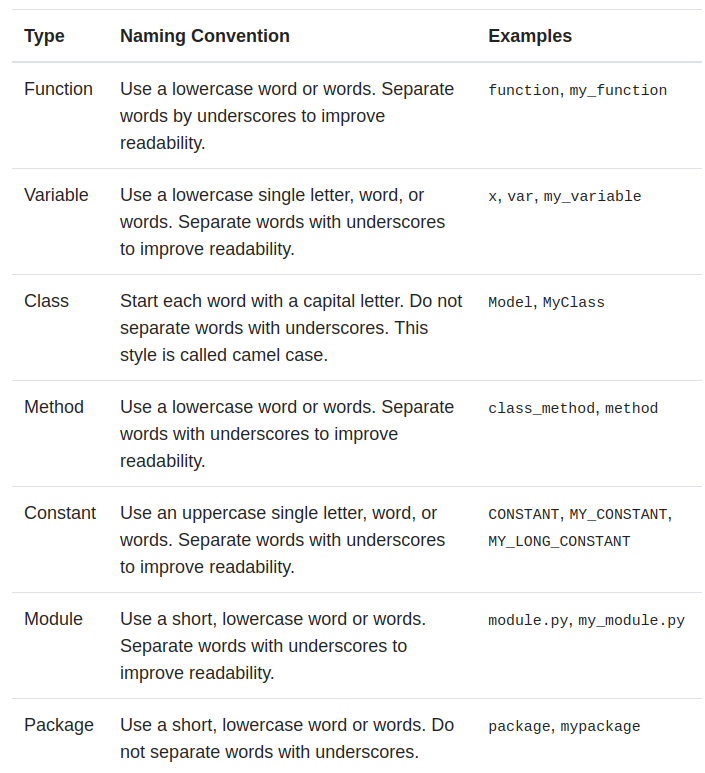This convention is used for declaring private variables, functions, methods and classes in a module. Anything with this convention are ignored in from module import *.

single_trailing_underscore_
This convention could be used for avoiding conflict with Python keywords or built-ins. You might not use it often.

This convention is used for special variables or methods (so-called “magic method”) such as__init__, ___len__. These methods provides special syntactic features or does special things. For example, __file___ indicates the location of Python file, ___eq___ is executed when a == b expression is excuted. A user of course can make custom special method, it is very rare case, but often might modify the some built-in special methods. (e.g. You should initialize the class with ___init___ that will be executed at first when a instance of class is created.)

## Files Operation

open a file & print line one by one

``````with open("file_path/dir", "w/r/a", encoding='utf8') as f:
print(line.split("car"), end=' ')
``````

open a file & read lines

``````with open("file_path/dir", "w/r/a", encoding='utf8') as f:
``````

open a file & write line one by one or all together

``````with open("file_path/dir", "w/r/a", encoding='utf8') as f:
...
f.write() # or
f.writelines()
``````

## Various Tricks

reverse a string

``````"This is a string"[::-1]
``````
``````'gnirts a si sihT'
``````

conditional assignement in one line (without ‘else’)

``````x, y = 0, 10
if y == 10: x = 5
x
``````
``````5
``````

concatenate 2 dictionnaries

``````i = {'a': 1, 'b': 2, 'c': 3}
j = {'d': 4, 'e': 5}
{**i, **j}
``````
``````{'a': 1, 'b': 2, 'c': 3, 'd': 4, 'e': 5}
``````

print the path of the ‘os’ module

``````import os
print(os)
``````
``````<module 'os' from '/usr/lib/python3.7/os.py'>
``````

conditional assignement in one line with ‘else’ case

``````y = 10
x = 'a' if y == 10 else 'b'
x
``````
``````'a'
``````
``````y = 10
x = 'a' if y != 10 else 'b'
x
``````
``````'b'
``````

compute the frequency of a list elements

``````lst = ['a', 'b', 'b', 'c', 'c', 'c']
freq_dict = {}

for i in lst:
if i not in freq_dict.keys():
freq_dict[i] = 1
freq_dict[i] += 1

freq_dict
``````
``````{'a': 2, 'b': 3, 'c': 4}
``````

get the help of a module function

``````from math import cosh
help(cosh)
``````
``````Help on built-in function cosh in module math:

cosh(x, /)
Return the hyperbolic cosine of x.
``````

retrieve most frequent element of a list

``````lst = ['a', 'b', 'b', 'c', 'c', 'c']
most_freq = max(set(lst), key=lst.count)
most_freq
``````
``````'c'
``````
``````def most_frequent(List):
return max(set(List), key = List.count)

most_frequent(['a', 'b', 'b', 'c', 'c', 'c'])
``````
``````'c'
``````

declare a Car & a Plane classes, create an instance of one of the two classes depending on an other value

``````class Car(object):
def __init__(self, value):
self.property = "car"
self.value = value

class Plane(object):
def __init__(self, param):
self.property = "plane"
self.param = param

y == 10
x = (Car if y == 10 else Plane)(22)
isinstance(x, Car)
``````
``````True
``````
``````z = (Car if y!= 10 else Plane)(33)
z.property, z.param
``````
``````('plane', 33)
``````

Tags:

Categories:

Updated: